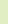[an error occurred while processing the directive]

# The Joy of Hex

Cisco certification candidates, particularly CCNA candidates, must master binary math. This includes basic conversions, such as binary-to-decimal and decimal-to-binary, as well as more advanced scenarios involving subnetting and VLSM.

There’s another conversion that might rear its ugly head on your Cisco exam, though, and that involves hexadecimal numbering.

Newcomers to hexadecimal numbering are often confused as to how a letter of the alphabet can possibly represent a number. Worse, they may be intimidated – after all, there must be some incredibly complicated formula involved with representing the decimal 11 with the letter “b”, right?

Wrong.

The numbering system we use every day, decimal, concerns itself with units of ten. Although we rarely stop to think of it this way, if you read a decimal number from right to left, the number indicates how many units of one, ten, and one hundred we have. That is, the number “15” is five units of one and one unit of ten. The number “289” is nine units of one, eight units of ten, and two units of one hundred. Simple enough!

Hex numbers are read much the same way, except the units here are units of one, 16, and 256. The number “15” in hex is read as having five units of one and one unit of sixteen. The number “289” in hex is nine units of one, eight units of sixteen, and two units of 256 (16 x 16).

Since hex uses units of sixteen, how can we possibly represent a value of 10, 11, 12, 13, 14, or 15? We do so with letters. The decimal “10” is represented in hex with the letter “a” the decimal 11 with “b” the decimal “12” with “c”, “13” with “d”, “14” with “e”, and finally, “15” with “f”. (CCNA candidates will remember that a MAC address of “ffff.ffff.ffff” is a Layer 2 broadcast.)

Practice Your Conversions For Exam Success
Now that you know where the letters fall into place in the hexadecimal numbering world, you’ll have little trouble converting hex to decimal and decimal to hex – if you practice.

How would you convert the decimal 27 to hex? You can see that there is one unit of 16 in this decimal that leaves 11 units of one. This is represented in hex with “1b” – one unit of sixteen, 11 units of one.

Converting the decimal 322 to hex is no problem. There is one unit of 256 that leaves 66. There are four units of 16 in 66 that leaves 2, or two units of one. The hex equivalent of the decimal 322 is the hex figure 142 – one unit of 256, four units of 16, and 2 units of 2.

Hex-to-decimal conversions are even simpler. Given the hex number 144, what is the decimal equivalent? We have one unit of 256, four units of 16, and four units of 4. This gives us the decimal figure 324.

What about the hex figure c2? We now know that the letter “c” represents the decimal number “12”. This means we have 12 units of 16, and two units of 2. This gives us the decimal figure 194.

Tips For Exam Day
Practice your binary and hexadecimal conversions over and over again before you take your CCNA exams. Binary math questions come in many different forms make sure you have practiced all of them before exam day. The number one reason CCNA candidates fail their exam is that they’re not prepared for the different types of binary math questions they’re going to be asked, and that they aren’t ready for hexadecimal questions at all.

You don’t have time to learn how to do in on exam day. You’ve got to be ready before you go into the exam room, and the only way to be ready is a lot of practice.

Finally, make sure you read the question carefully. You’ve got hex, decimal, and binary numbers to concern yourself with on your CCNA exams. Make sure you give Cisco the answer in the format they’re looking for.Sponsors